### 揭秘”智能定投“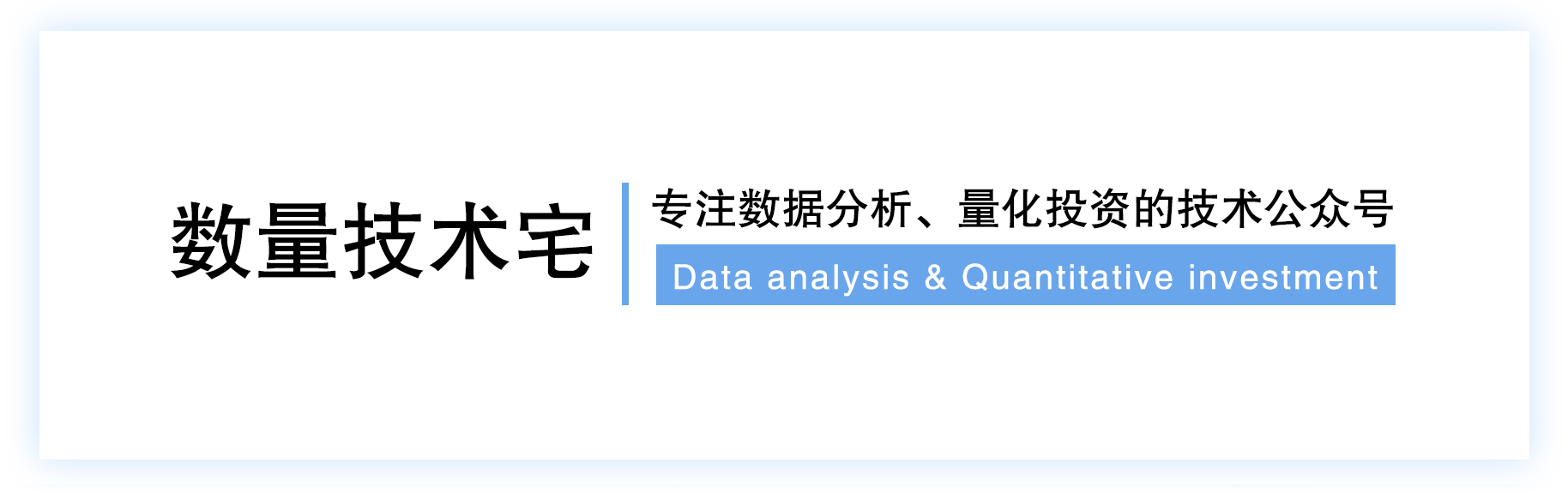## 什么是智能定投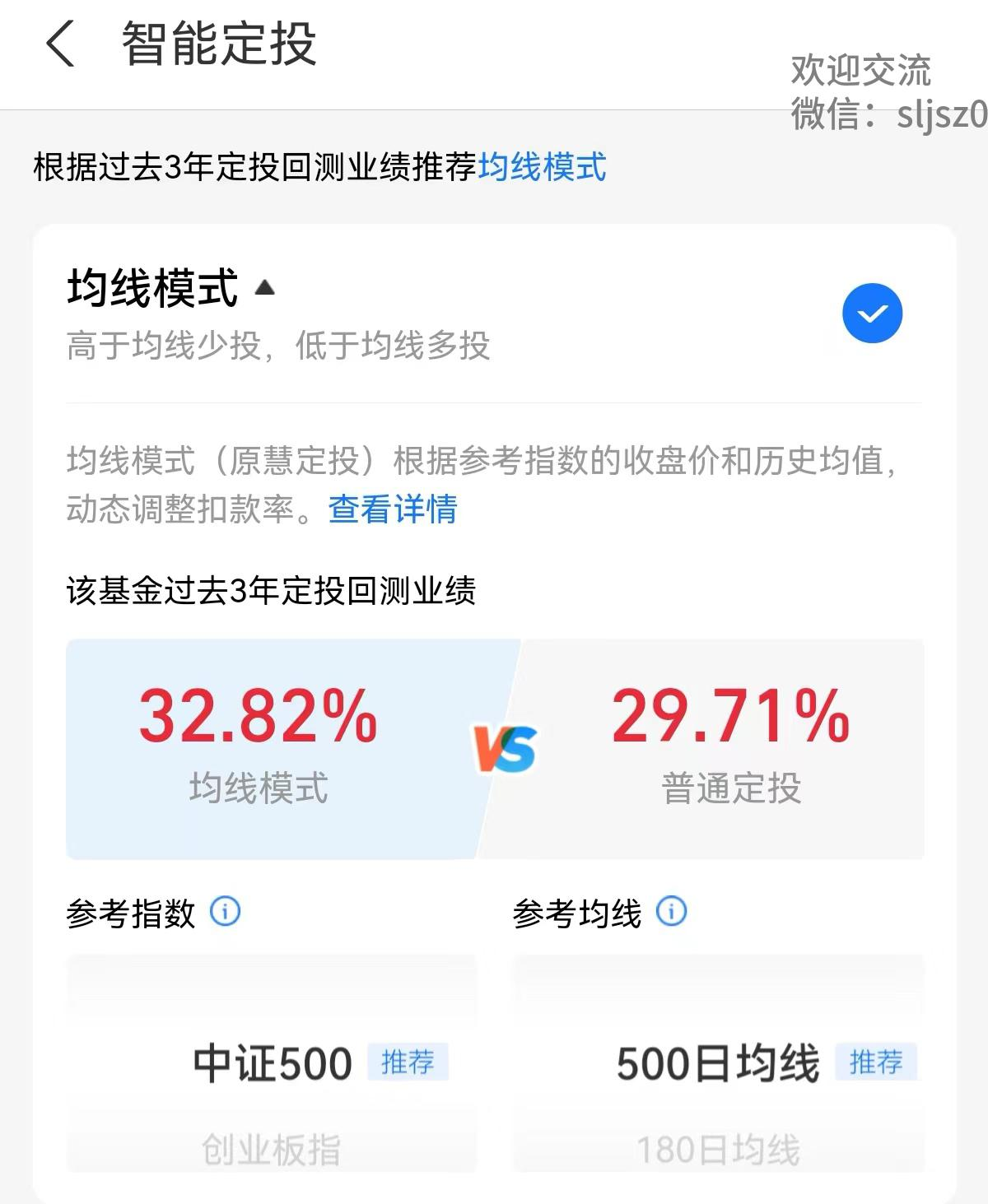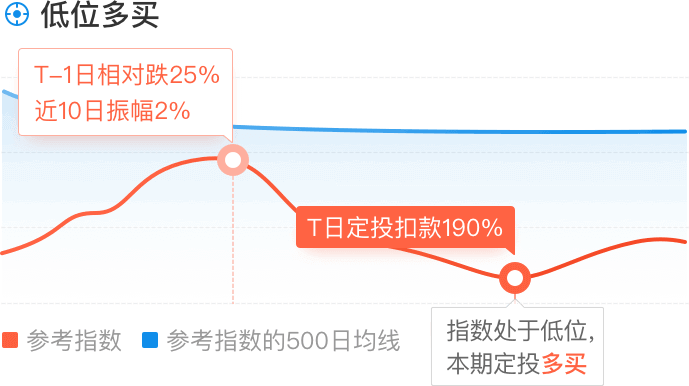## Python实现智能定投策略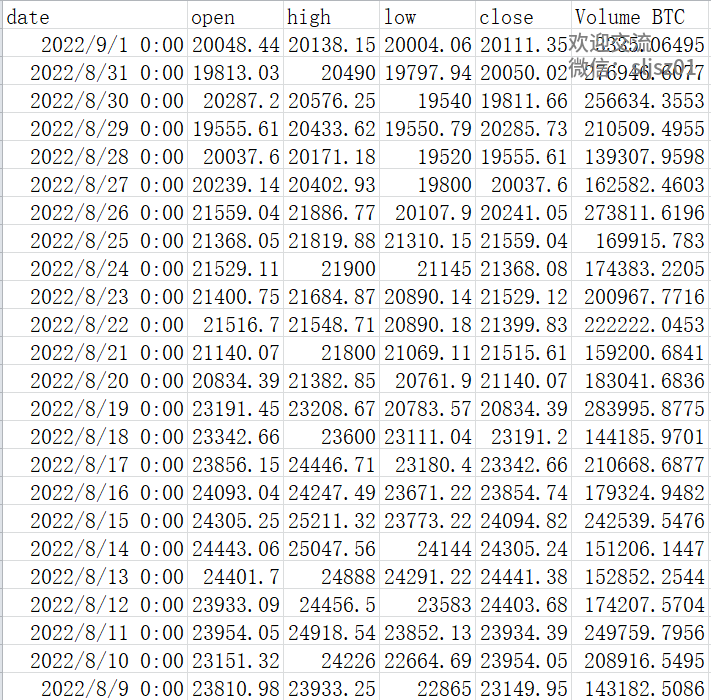```adj_1 = 0.4

```condition1 = XXX
df.loc[condition1, 'invest_val'] = base_invest_fun * (1 - adj_1)

condition2 = XXX
df.loc[condition2, 'invest_val'] = base_invest_fun * (1 - adj_2)

condition3 = XXX
df.loc[condition3, 'invest_val'] = base_invest_fun * (1 + adj_1)

condition4 = XXX
df.loc[condition4, 'invest_val'] = base_invest_fun * (1 + adj_2)```

```fig, ax1 = plt.subplots()
ax1.plot(df['date'], df['net_value'], 'r-', label='Market Value')
ax1.plot(df['date'], df['total_invest_val'], 'y-', label='My Total Cost')
ax1.set_xlabel("year")
ax1.set_title("BTC 定投")
plt.legend(loc='best')
plt.show()```

## 典型标的智能定投效果

BTC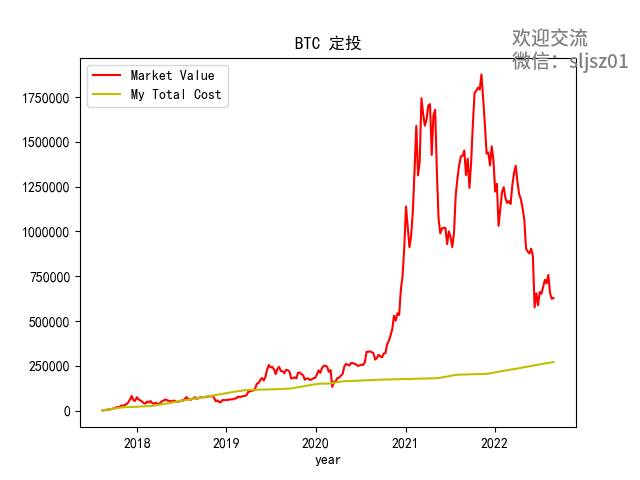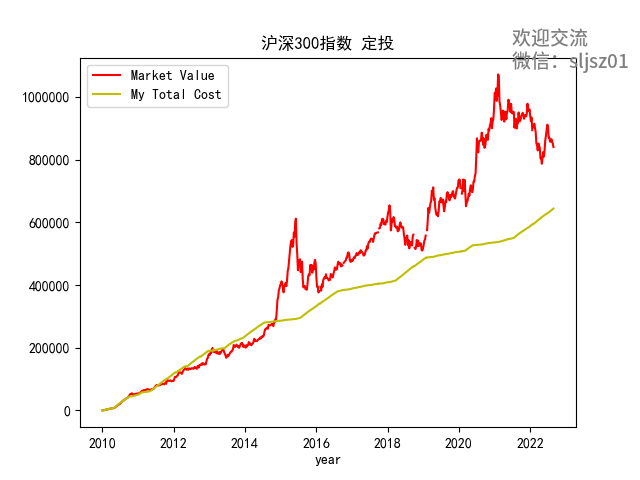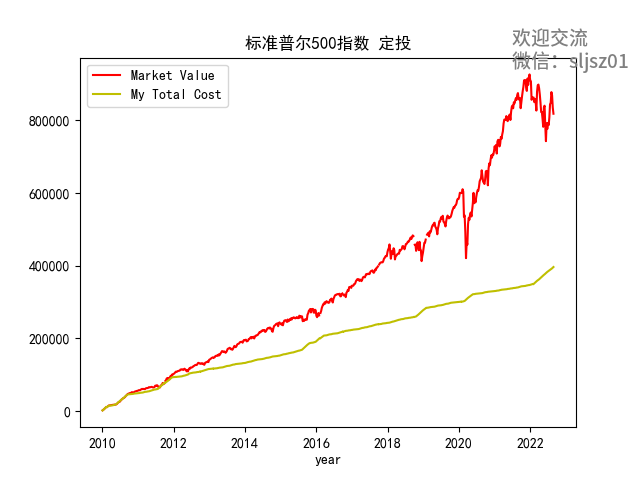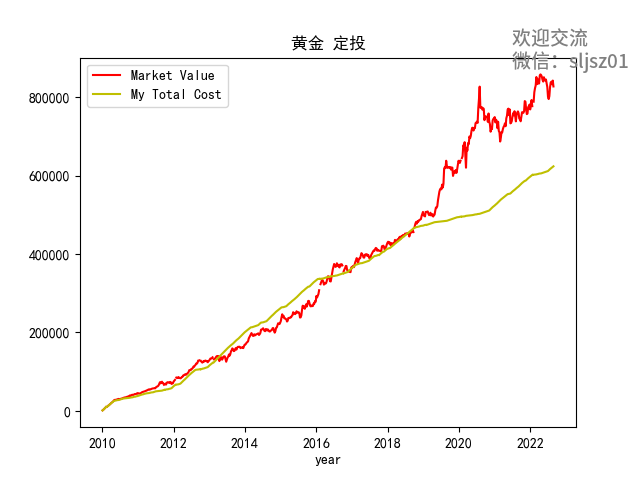## 往期干货分享推荐阅读

Omega System Trading and Development Club内部分享策略Easylanguage源码

AMA指标原作者Perry Kaufman 100+套交易策略源码分享

【数量技术宅|金融数据分析系列分享】为什么中证500（IC）是最适合长期做多的指数

【数量技术宅|金融数据分析系列分享】如何正确抄底商品期货、大宗商品

【数量技术宅|量化投资策略系列分享】股指期货IF分钟波动率统计策略

【数量技术宅 | Python爬虫系列分享】实时监控股市重大公告的Python爬虫